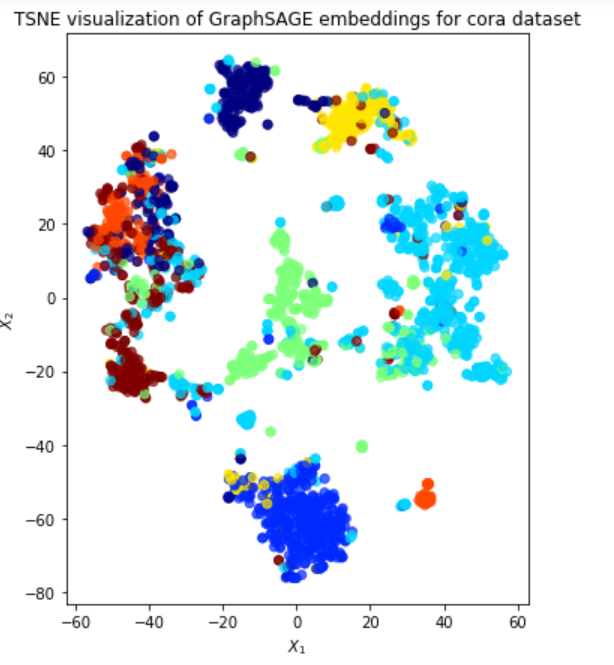# Using GraphSAGE to Learn Paper Embeddings in CORA

Source: Deep Learning on Medium# Introduction

CORA is a dataset of academic papers of seven different classes. It contains the citation relations between the papers as well as a binary vector for each paper that specifies if a word occurs in the paper. Thus, CORA contains both content-based features for each paper and relationship features between the papers. We can model these features with a network where each paper is represented by a node that carries the content-based features and citations are represented with edges.

With the graph model, we can use geometric deep learning approaches to learn embeddings for each paper. In this story, we use GraphSAGE.

GraphSAGE is an unsupervised node embedding algorithm, known for its success on large graphs. It can utilize node features and node relations to learn vectors for each node that represent the neighborhood structures in the graph. To read more on GraphSAGE you can refer to the story in the link.

To implement GraphSAGE, we use a Python library stellargraph which contains off-the-shelf implementations of several popular geometric deep learning approaches, including GraphSAGE. The installation guide and documentation of stellargraph can be found here. Additionally, the code used in this story is based on the example in the library’s GitHub repository .

# Graph Creation

stellargraph library uses StellarGraph object to represent graphs. Luckily, we can initialize a StellarGraph object quite easily from a networkx graph. So, we create a networkx graph by treating links in CORA as an edge list. Note that this creates the necessary nodes automatically. We then add content-based features to each node by parsing cora.content file and indexing each unique word from 1 to the number of unique words, 1433. We also store these features separately in a variable named node_features. We use these features to create a StellarGraph object.

`cora_dir = './cora/'edgelist = pd.read_csv(cora_dir+'cora.cites, sep='\t', header=None, names=['target', 'source'])edgelist['label'] = 'cites' # set the edge typeGnx = nx.from_pandas_edgelist(edgelist, edge_attr='label')nx.set_node_attributes(Gnx, 'paper', 'label')# Add content featuresfeature_names = ["w_{}".format(ii) for ii in range(1433)]column_names = feature_names + ['subject']node_data = pd.read_csv(data_dir+'cora.content), sep='\t', header=None, names=column_names)node_features = node_data[feature_names]# Create StellarGraph objectG = sg.StellarGraph(Gnx, node_features=node_features)`

Thanks to networkx and pandas, we loaded CORA to a networkx graph and then created a StellarGraph object in just a couple of lines. We can now use the created object to implement GraphSAGE.

# Model Training

To implement GraphSAGE, we will use the modules inside stellargraph. stellargraph contains an UnsupervisedSampler class to sample a number of walks of given length from the graph. We also use a GraphSAGELinkGenerator to generate the edges needed in the loss function. Note that GraphSAGE exploits the link prediction task to create similar embeddings for adjacent nodes. The generator creates the edges from the sampled walks.

`# Specify model and training parametersnodes = list(G.nodes())number_of_walks = 1length = 5batch_size = 50epochs = 4num_samples = [10, 5]unsupervised_samples = UnsupervisedSampler(G, nodes=nodes, length=length, number_of_walks=number_of_walks)train_gen = GraphSAGELinkGenerator(G, batch_size, num_samples) .flow(unsupervised_samples)`

Having generated the walks and created a link generator, we now define and build GraphSAGE model. The built object returns input/output placeholders for us to fill later on. Based on the output placeholder, we add a prediction layer with sigmoid activation, since link prediction is a binary classification problem.

`layer_sizes = [50, 50]graphsage = GraphSAGE(layer_sizes=layer_sizes, generator=train_gen, bias=True, dropout=0.0, normalize='l2')# Build the model and expose input and output sockets of graphsage, # for node pair inputsx_inp, x_out = graphsage.build()prediction = link_classification(output_dim=1, output_act='sigmoid', edge_embedding_method='ip')(x_out)`

Here comes the cool part! We can use the prediction layer and input placeholder to create a keras model and train it to learn the embeddings! Thanks to the fact that stellargraph uses keras’ layers inside its code, each implemented graph algorithm is compatible with keras. Therefore, we can use keras’ utilities such as loss tracking and hyper-parameter tuning in a native way.

`model = keras.Model(inputs=x_inp, outputs=prediction)model.compile( optimizer=keras.optimizers.Adam(lr=1e-3), loss=keras.losses.binary_crossentropy, metrics=[keras.metrics.binary_accuracy],)history = model.fit_generator( train_gen, epochs=epochs, verbose=1, use_multiprocessing=False, workers=4, shuffle=True,)`

We have trained an edge prediction model to learn paper embeddings. We can use this model to create an embedding generator, given a node. To do so, we create another keras model named embedding_model and create a GraphSAGENodeGenerator object. Combining these two, we can obtain the embeddings of each paper in CORA!

`x_inp_src = x_inp[0::2]x_out_src = x_outembedding_model = keras.Model(inputs=x_inp_src, outputs=x_out_src)node_ids = node_data.indexnode_gen = GraphSAGENodeGenerator(G, batch_size,num_samples)  .flow(node_ids)node_embeddings = embedding_model.predict_generator(node_gen, workers=4, verbose=1)`

To validate that these embeddings are meaningful, we plot the resulting embeddings on 2D using TSNE. We color each paper by its class in the dataset and observe that papers of the class are grouped together in the plot. Given that we did not exploit the class labels during training, this is an amazing result!

# Conclusion

In this story, we run GraphSAGE on CORA dataset and learned paper embeddings. We utilized stellargraph library since it presents an easy-to-use interface that is also compatible with networkx and keras libraries. Thanks to stellargraph, we can try out different geometric different learning approaches quickly and compare their results. This renders stellargraph an ideal starting point to create baselines and to try network-based approaches on the problem at hand.

## References

 CORA

 stellargraph GitHub